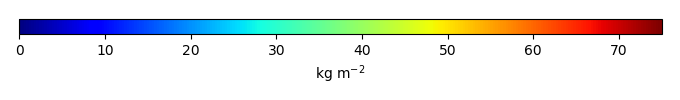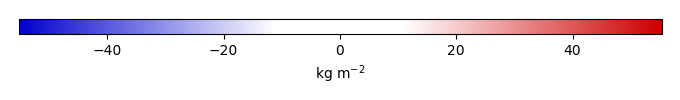# Mean State

Period Mean (original grids) [Pg]
Model Period Mean (intersection) [Pg]
Model Period Mean (complement) [Pg]
Benchmark Period Mean (intersection) [Pg]
Benchmark Period Mean (complement) [Pg]
Bias [kg m-2]
Bias Score 
Spatial Distribution Score 
Overall Score 
Benchmark [-] 76.5
CLM5PHSOFF [-] 66.9 55.6 11.8 76.1 0.362 -7.37 0.57 0.58 0.58
CLM5PHSON [-] 101. 90.3 10.0 76.1 0.362 6.17 0.53 0.30 0.42
Period Mean (original grids) [Pg]
Model Period Mean (intersection) [Pg]
Model Period Mean (complement) [Pg]
Benchmark Period Mean (intersection) [Pg]
Benchmark Period Mean (complement) [Pg]
Bias [kg m-2]
Bias Score 
Spatial Distribution Score 
Overall Score 
Benchmark [-] 113.
CLM5PHSOFF [-] 74.8 35.0 37.8 113. 0.00185 -16.4 0.50 0.53 0.52
CLM5PHSON [-] 133. 70.5 59.6 113. 0.00185 -9.00 0.56 0.83 0.69
Period Mean (original grids) [Pg]
Model Period Mean (intersection) [Pg]
Model Period Mean (complement) [Pg]
Benchmark Period Mean (intersection) [Pg]
Benchmark Period Mean (complement) [Pg]
Bias [kg m-2]
Bias Score 
Spatial Distribution Score 
Overall Score 
Benchmark [-] 12.2
CLM5PHSOFF [-] 63.0 2.73 61.5 12.2 0.00 -9.64 0.47 0.63 0.55
CLM5PHSON [-] 91.8 5.31 88.9 12.2 0.00 -6.98 0.55 0.82 0.68
Period Mean (original grids) [Pg]
Model Period Mean (intersection) [Pg]
Model Period Mean (complement) [Pg]
Benchmark Period Mean (intersection) [Pg]
Benchmark Period Mean (complement) [Pg]
Bias [kg m-2]
Bias Score 
Spatial Distribution Score 
Overall Score 
Benchmark [-] 97.3
CLM5PHSOFF [-] 87.0 76.4 11.6 97.2 0.0996 -6.59 0.61 0.73 0.67
CLM5PHSON [-] 119. 110. 10.0 97.2 0.0996 4.55 0.54 0.39 0.47
Period Mean (original grids) [Pg]
Model Period Mean (intersection) [Pg]
Model Period Mean (complement) [Pg]
Benchmark Period Mean (intersection) [Pg]
Benchmark Period Mean (complement) [Pg]
Bias [kg m-2]
Bias Score 
Spatial Distribution Score 
Overall Score 
Benchmark [-] 5.12
CLM5PHSOFF [-] 39.5 3.01 35.6 5.12 0.00 -7.97 0.61 0.64 0.63
CLM5PHSON [-] 44.2 3.46 40.1 5.12 0.00 -6.30 0.64 0.62 0.63
Period Mean (original grids) [Pg]
Model Period Mean (intersection) [Pg]
Model Period Mean (complement) [Pg]
Benchmark Period Mean (intersection) [Pg]
Benchmark Period Mean (complement) [Pg]
Bias [kg m-2]
Bias Score 
Spatial Distribution Score 
Overall Score 
Benchmark [-] 475.
CLM5PHSOFF [-] 864. 275. 611. 474. 1.51 -8.82 0.53 0.39 0.46
CLM5PHSON [-] 1.06e+03 433. 622. 474. 1.51 -1.41 0.53 0.64 0.59
Period Mean (original grids) [Pg]
Model Period Mean (intersection) [Pg]
Model Period Mean (complement) [Pg]
Benchmark Period Mean (intersection) [Pg]
Benchmark Period Mean (complement) [Pg]
Bias [kg m-2]
Bias Score 
Spatial Distribution Score 
Overall Score 
Benchmark [-] 116.
CLM5PHSOFF [-] 73.1 60.2 12.4 116. 0.373 -9.15 0.51 0.72 0.61
CLM5PHSON [-] 123. 115. 7.60 116. 0.373 0.749 0.49 0.62 0.56
Period Mean (original grids) [Pg]
Model Period Mean (intersection) [Pg]
Model Period Mean (complement) [Pg]
Benchmark Period Mean (intersection) [Pg]
Benchmark Period Mean (complement) [Pg]
Bias [kg m-2]
Bias Score 
Spatial Distribution Score 
Overall Score 
Benchmark [-] 0.0131
CLM5PHSOFF [-] 20.3 0.00162 19.6 0.0131 0.00 -5.18 0.42 4.0e-22 0.21
CLM5PHSON [-] 32.2 0.0178 31.5 0.0131 0.00 2.19 0.69 2.5e-23 0.35
Period Mean (original grids) [Pg]
Model Period Mean (intersection) [Pg]
Model Period Mean (complement) [Pg]
Benchmark Period Mean (intersection) [Pg]
Benchmark Period Mean (complement) [Pg]
Bias [kg m-2]
Bias Score 
Spatial Distribution Score 
Overall Score 
Benchmark [-] 46.1
CLM5PHSOFF [-] 45.7 21.6 24.5 46.1 0.00725 -11.9 0.51 0.32 0.41
CLM5PHSON [-] 75.2 36.0 39.5 46.1 0.00725 -4.90 0.56 0.58 0.57
Period Mean (original grids) [Pg]
Model Period Mean (intersection) [Pg]
Model Period Mean (complement) [Pg]
Benchmark Period Mean (intersection) [Pg]
Benchmark Period Mean (complement) [Pg]
Bias [kg m-2]
Bias Score 
Spatial Distribution Score 
Overall Score 
Benchmark [-] 0.232
CLM5PHSOFF [-] 7.06 0.0855 7.54 0.232 0.00 -3.26 0.44 0.48 0.46
CLM5PHSON [-] 11.5 0.289 11.9 0.232 0.00 0.143 0.53 0.81 0.67

# Temporally integrated period mean

BENCHMARK MEANMODEL MEANBIASBIAS SCORESPATIAL TAYLOR DIAGRAMMODEL COLORS# Spatially integrated regional mean

MODEL COLORS# All Models

BenchmarkCLM5PHSOFFCLM5PHSON# Data Information

Approach: I just read the data from the original data file and converted the latitude arrange from original 90N->90S to 90S->90N. The unit was convert from original 0.1 KgC/m2 to KgC/m2

Temporal resolution: yearly

General information: This product was derived from Northern Circumpolar Soil Carbon Database V2.2 (NCSCDv22) in top 1m.

Spatial resolution: 0.5x0.5 degree